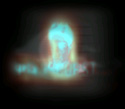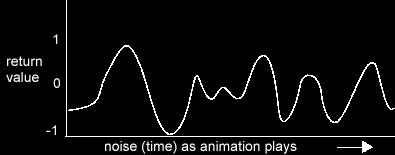page 1 2 3 - the shaker - MAYA TUTORAL 2DESTABILISATION     Early on in my design stage, I realised that a camera which was shaking might also be subject to a second offset of movement over longer periods of time.      The best example is too imagine someone holding a camera and that person is standing on something which is vibrating or is in a vehicle going over a bumpy road. Through the lens you will see lots of violent shake but also, over time, there will be another offset. The person holding the camera will also have his limbs and body moved locally over time as a result of the initial shaking.      If a camera was firmly attached to a rollercoaster then there would be no secondary movement as a result of the initial shake (unless the bracket fixing the camera to the coaster was loose or flexible). In this example, Destabilisation would be of no use.      The Destabilise property represents this second random movement and is created by using the 'noise (time)' random number generator      This returns a value between -1 and 1 each time the expression executes as an animation plays. Because time increases in fine increments, the values returned increase and decrease in smooth, yet random, patterns. If you were to chart the values returned over a period of time, they might occur as in this figure:Again, the expression for this destabilisation offset is applied to each translation and rotation channel. As we are dealing with just one single node for the whole utility, the expression has to be combined with the shake property, so we end up with:      the_shaker.tx = ( gauss ( ( rand ( 0 , 1 ) ) ) )   +     noise (time)      In reality, just copying this same function to each channel will create the same movement for each channel so you need to offset the time parameter so that xyz trans and rotation is different at any point in time:        the_shaker.tx = ( gauss ( ( rand ( 0 , 1 ) ) ) )   +     noise (time - 50)  the_shaker.ty = ( gauss ( ( rand ( 0 , 1 ) ) ) )   +     noise (time)  the_shaker.tz = ( gauss ( ( rand ( 0 , 1 ) ) ) )   +     noise (time + 70)     the_shaker.rx = ( gauss ( ( rand ( 0 , 1 ) ) ) )   +     noise (time - 30)  the_shaker.ry = ( gauss ( ( rand ( 0 , 1 ) ) ) )   +     noise (time - 70)  the_shaker.rz = ( gauss ( ( rand ( 0 , 1 ) ) ) )   +     noise (time + 100)        the figures added or subtracted to the time are arbitrary - they just need to be different for each channel but far enough apart so one channel cannot be seen copying another.      So in summary, the noise(time) random generator will add a new offset of movement to the shaker node at each frame, but an offset which is based upon a smooth curve which changes gradually over time. It is worth noting that this Destabilisation movement is visually quite pleasing and can be used for all sorts of interesting effects. It has a very gentle quality which would serve a number of simulations found in everyday life.              WANDER   If an object is the subject of a lot of shaking or vibration, it is quite likely that a third type of movement - wander - would be seen. That is, the object would tend to actually move its overall position as a result of the initial shake.      During an earthquake, the pair of shoes you left by the front door would vibrate and shake about but also, after a while, they may well end up in the living room. This is what I call Wander.      Wander is simulated simply by using the 'rand (x,y)' function which returns a random number between x and y at every frame but it is applied cumulatively to the translation and rotation channels. Remember that the Shake property was driven by:      x translation = gaussian random number of another random number between x and y      so, at each frame there is an offset of movement but the node still remains within the same range of values. It remains anchored to a specific area in space.      By applying random movement cumulatively:      x translation = x translation + random number between -1 and 1       random movement will be added to the existing position/rotation (or subtracted if the random number is less than 0 ). The node can achieve position and rotation way outside the offsets dictated by shake and destabilisation. Visually, a linked object will drift or wander.      Wander is only really applicable to translation so the expression is applied to the tx ty tz channels only:      the_shaker.tx =   the_shaker.tx   +   rand (-1,1)      For the moment, let us assume that this expression is combined with the other 2 properties to give:      the_shaker.tx =  ( gauss ( ( rand ( 0 , 1 ) ) ) )   +     noise (time - 50)    +  the_shaker.tx   +   rand (-1,1)      In reality, we have to use a lttle trick to allow for occasions where the wander intensity is zero and the animation should not include a cumulative addition of its previous value (+ the shaker.tx). More on this later.              JITTER     Jitter is the simplest property of the four. It is the same as shake but does not use the gaussian distribution. It just creates an offset of movement and/or rotation with the  rand (x,y) function:      the_shaker.tx =  rand (-1,1)      Again, this is combined with the other 3 properties:      the_shaker.tx =   gauss (( rand (0,1))) + noise (time - 50) + the_shaker.tx + rand (-1,1)  +  rand (-1,1)      Jitter was incorporated more as an after-thought in case the user needed to have a more jittery sort of shake that was purely random. In practice, a small amount added to the main shake seems to work well.        Go to the next page to find out how to integrate all the controls for the shaker.